## Artificial Neural Networks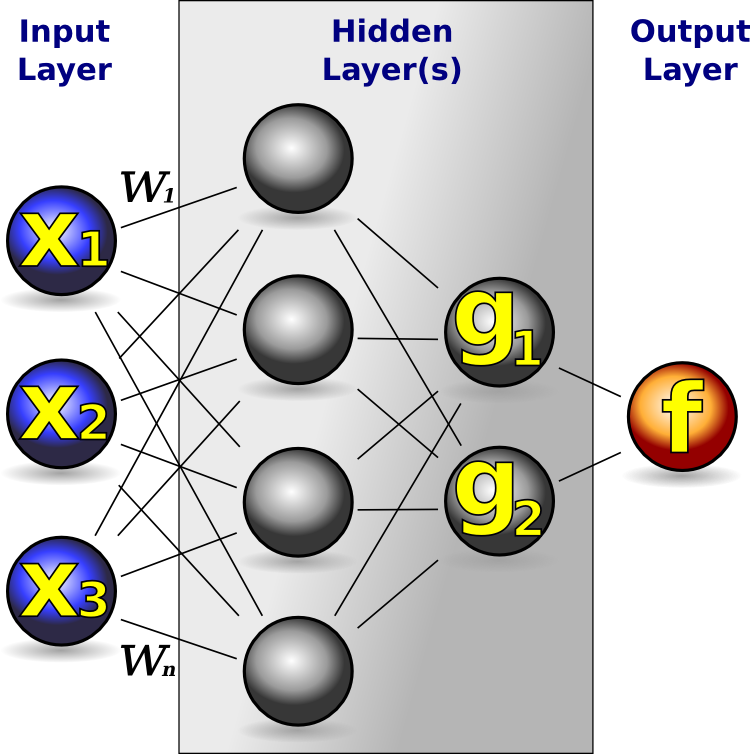Artificial Neural Networks (ANNs) have multiple representational forms. The most common are the mathematical (equation 3.2) and graphical form (figure 3.2). For each Artificial Neuron, the mathematical form consists of a function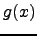of the input vector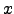, where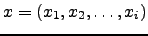. Each input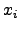is weighted according to its weight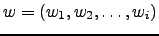.is the post-processing function that is finally applied. This results in the following equation for a single neuron: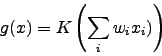(3.1)

Neural Networks consist of multiple artificial neurons like these. The output of one neuron is connected to the input of another neuron. Mathematically,(the result of equation 3.1) is an input to a neuron with function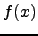:(3.2)

To introduce non-linearity, a hyperbolic tangent or sigmoid (S-shaped) function is commonly used for. Non-linearity is deliberately analogous to biological neurons, and responsible for its versatile information processing properties.

A reason for its popularity [Wik07b] in neural networks is because the sigmoid function satisfies the differential equation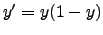. The right hand side is a low order polynomial. Furthermore, the polynomial has factors y and, both of which are simple to compute. Given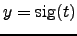at a particular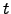, the derivative of the sigmoid function at thatcan be obtained by multiplying the two factors together. These relationships result in simplified implementations of artificial neural networks with artificial neurons.

Erik de Bruijn 2007-10-19